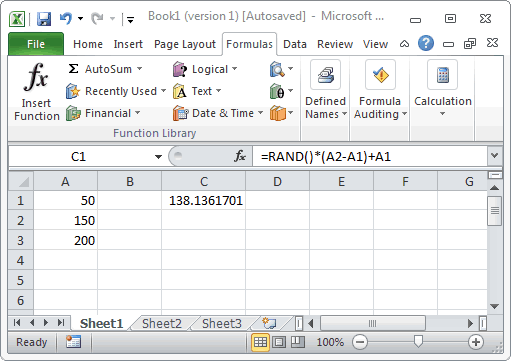# How to Apply Rand Function in your Excel Worksheet

Learn how to use the Excel RAND function with syntax and examples.Description

The Microsoft Excel RAND function returns a random number that is greater than or equal to 0 and less than 1. The RAND function returns a new random number each time your spreadsheet recalculates.

To create a random number between a range, you might also want to look at the RANDBETWEEN function.

## Syntax

The syntax for the Microsoft Excel RAND function is:

`RAND( )`

### Parameters or Arguments

There are no parameters or arguments for the RAND function.

## Note

To create a random number between two numbers, you can use the following formula:

`=RAND()*(b-a)+a`

Where a is the smallest number and b is the largest number that you want to generate a random number for. Please note, that this formula will never generate a number at the highest end of the range.

`=RAND()*(25-10)+10`

The formula above would generate a random number between 10 and 24.9999999.

## Applies To

The RAND function can be used in the following versions of Microsoft Excel:

• Excel 2013, Excel 2011 for Mac, Excel 2010, Excel 2007, Excel 2003, Excel XP, Excel 2000

## Type of Excel Function

The RAND function can be used in Microsoft Excel as the following type of function:

• Worksheet function (WS)

## Example (as Worksheet Function)

Let’s look at some Excel RAND function examples and explore how to use the RAND function as a worksheet function in Microsoft Excel:Based on the spreadsheet above, the following Excel RAND examples would return:

 =RAND()*(A2-A1)+A1 would return a random number (between 50 and 149.9999999) =RAND()*(A3-A1)+A1 would return a random number (between 50 and 199.9999999) =RAND()*(200-100)+100 would return a random number (between 100 and 199.9999999) =INT(RAND()*(200-100)+100) would return a random number (between 100 and 199, integer value)

Credit: http://www.techonthenet.com/excel/formulas/rand.php